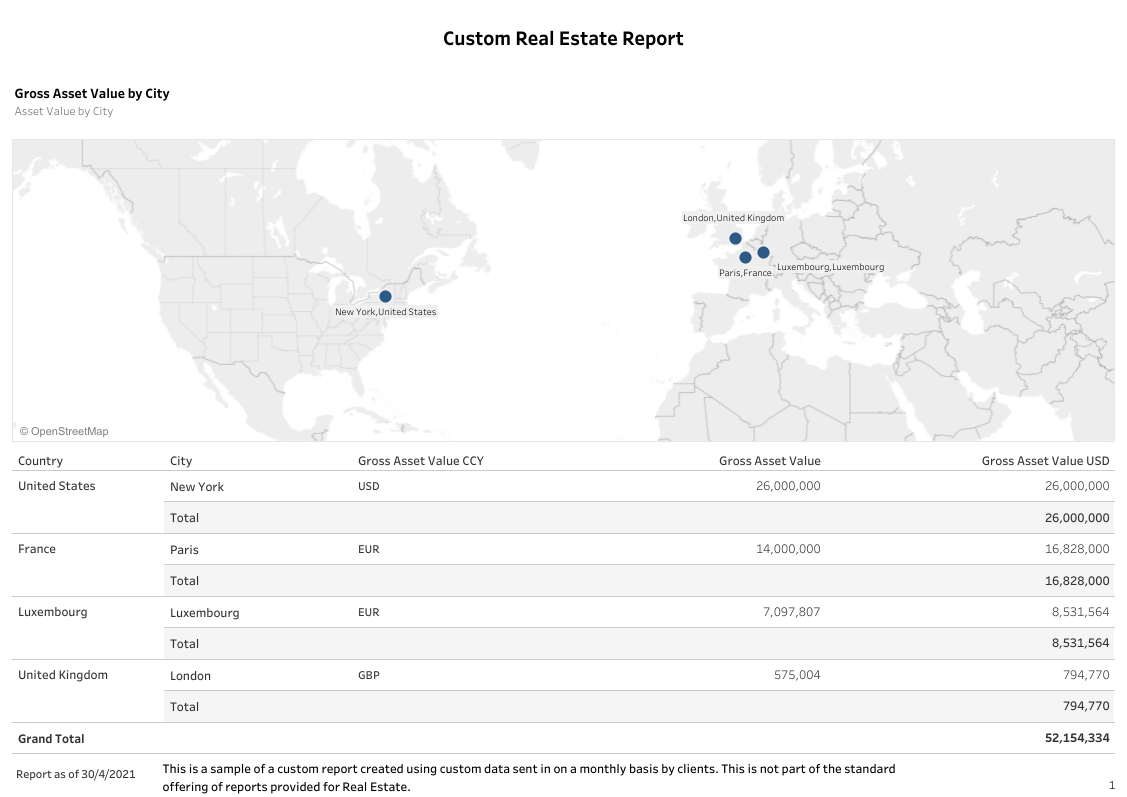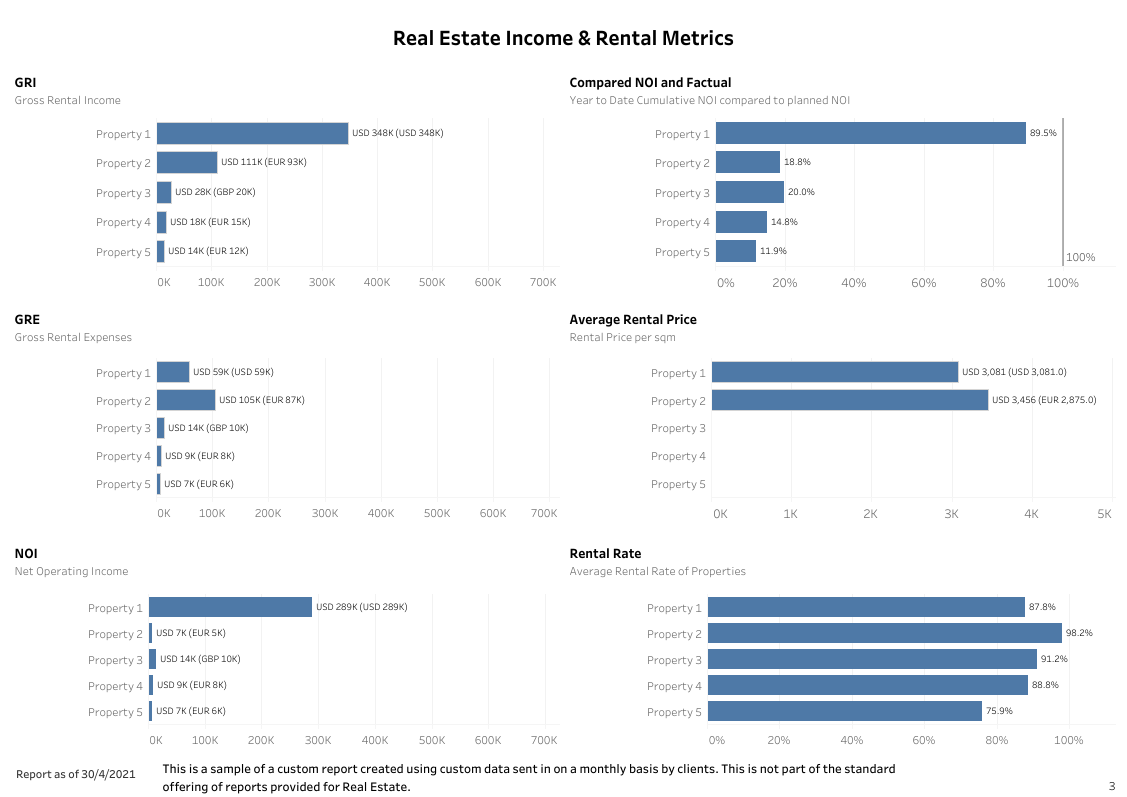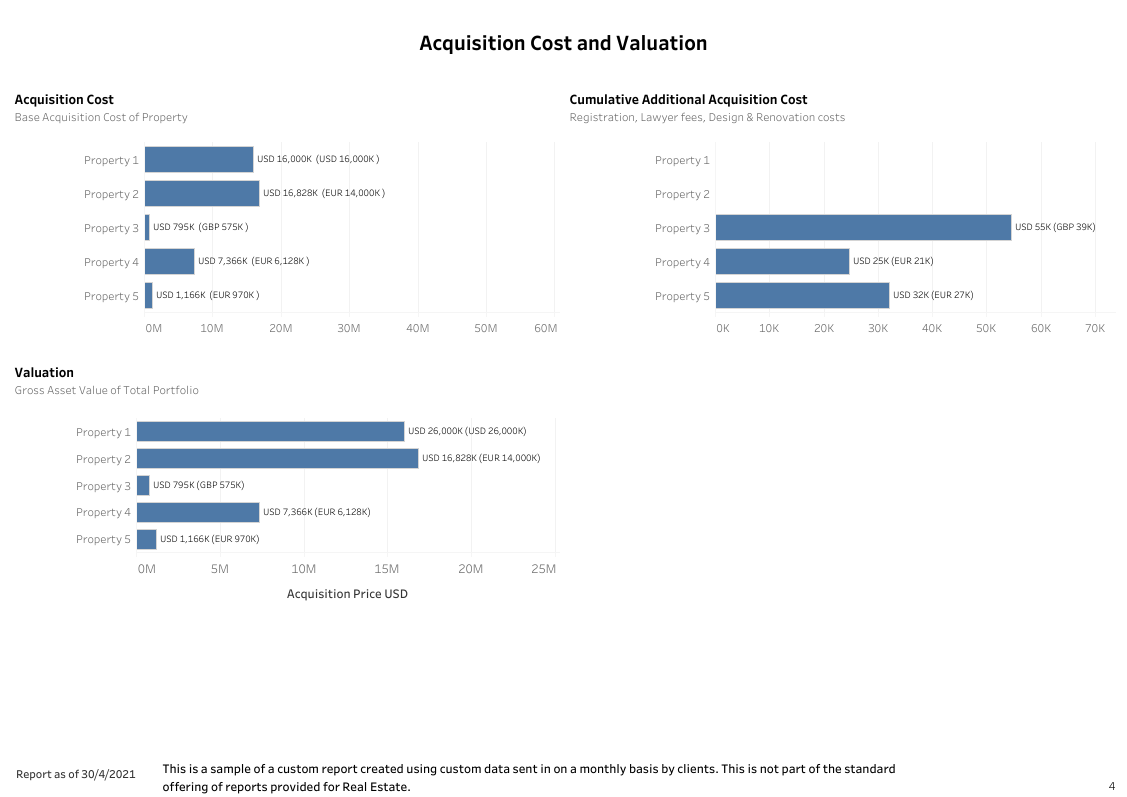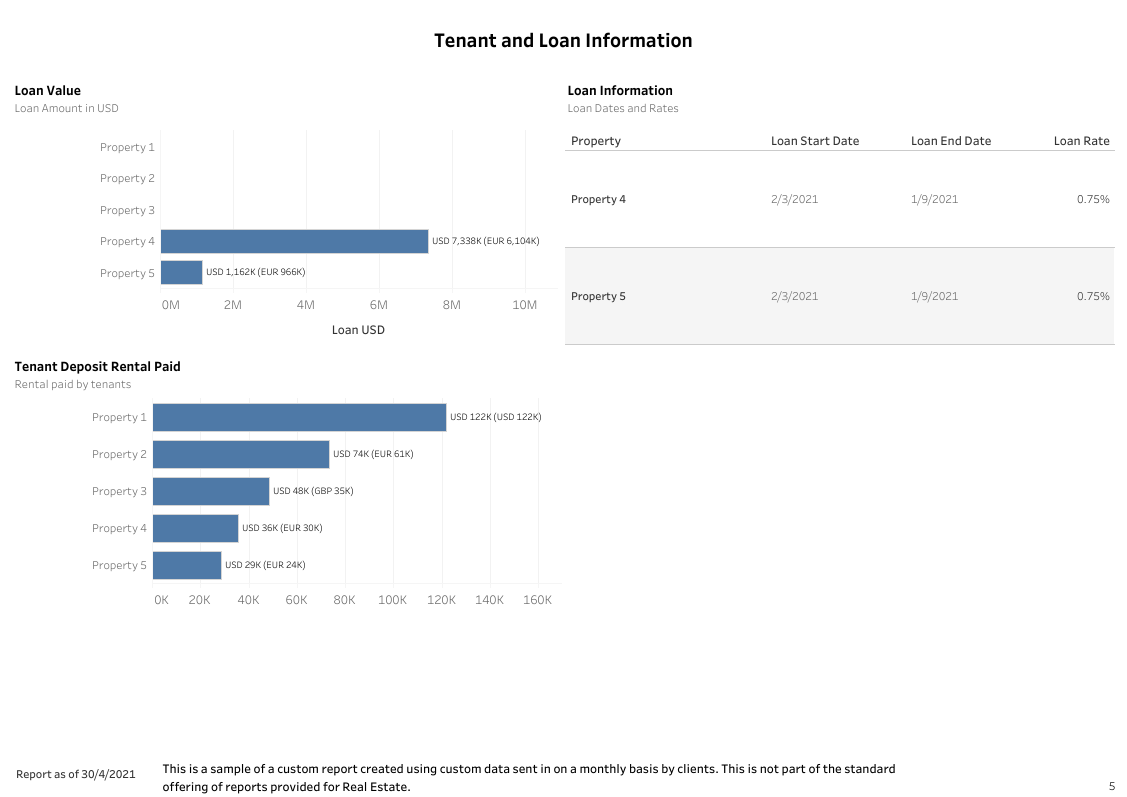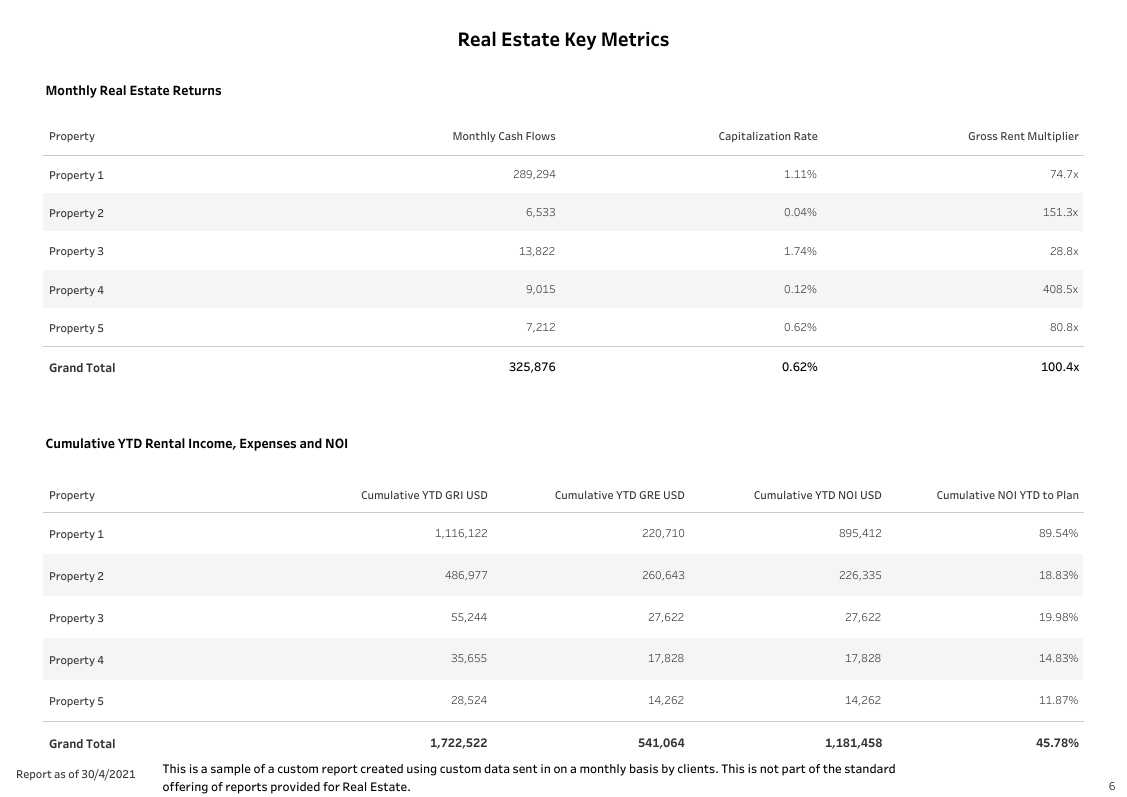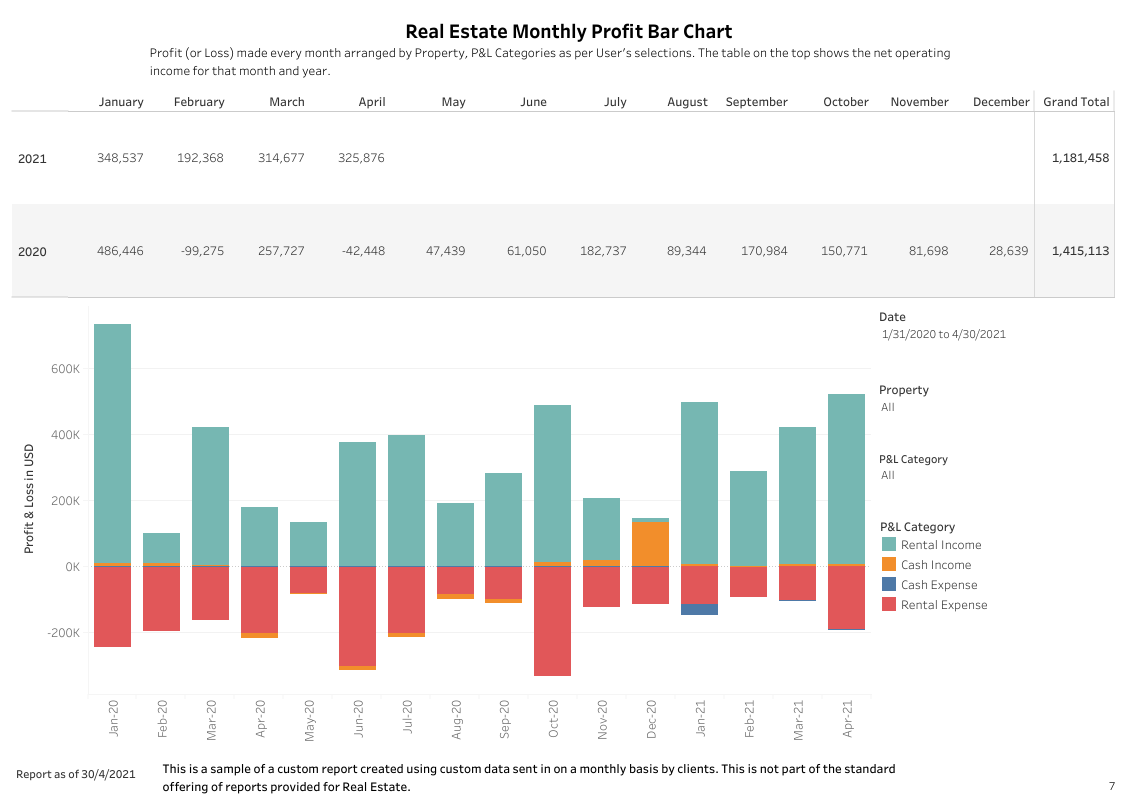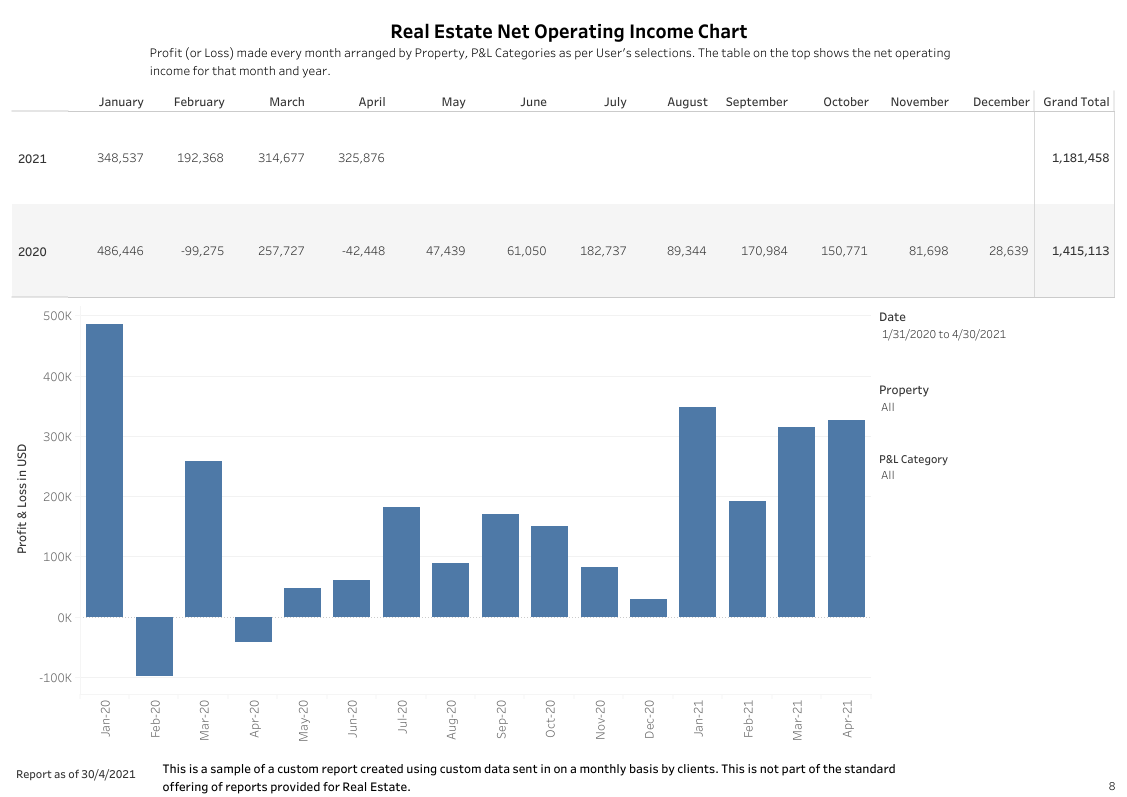# Metrics usually tracked for Real Estate

### Net Operating Income

Net operating income (NOI) is one of the key metrics of the financial analysis. It is the total income the property generates (after all expenses), not including debt service costs (loan costs).

### Cash Flow

Cash flow is the total profit you will see at the end of the year from the property. To determine the cash flow, simply subtract the total expenses from the total income. The higher your debt service payments (the larger your loan, the higher your interest rate, or the shorter your amortization period), the smaller your cash flow.

### Capitalization Rate

The capitalization rate (Cap Rate) is used determine what a property is worth. It considers vacancy, credit losses, other income, and operating expenses. Because the Cap Rate is independent of the buyer and the financing, it is the purest indication of the return a property will generate.

### Cash on Cash Return

Cash-on-cash (COC) return is directly related to the amount of cash you put down on the investment. It is the equivalent measure of how much return you would make for each dollar you put into the property.

### Internal Rate of Return

Internal rate of return (IRR) for an investment is the percentage rate earned on each dollar invested for each period it is invested. IRR is also another term people use for interest or yield.

### Net Present Value

Net present value (NPV) is an investment measure that shows whether the investment is achieving a target yield at a given initial investment. It also quantifies the adjustment to the initial investment needed to achieve the target yield, assuming everything else remains the same.

### Gross Rent Multiplier

is an indicator whereby price is divided by gross rents. Typically, a GRM in a great location goes between a range of 7-8.

# Sample Dashboards

Canopy is able to support all sorts of Dashboards. Some of the popular ones are below